# NCERT Solutions for Class 9 Science Work and Energy part 2

NCERT solutions for Science  Work and Energy## NCERT Class 9 Science Chapter wise Solutions

• 01 –  Matter in Our Surroundings
• 02 – Is Matter Around us Pure
• 03 – Atoms and Molecules
• 04 – Structure of the Atom
• 05 – The Fundamental Unit of Life
• 06 – Tissues
• 07 – Diversity in Living Organisms
• 08 – Motion
• 09 – Force and Laws of Motion
• 10 – Gravitation
• 11 – Word and Energy
• 12 – Sound
• 13 – Why Do We Fall Ill
• 14 – Natural Resources
• 15 – Improvement in Food Resources

## NCERT Solutions for Class 9 Science Work and Energy part 2

###### 1. Look at the activities listed below. Reason out whether or not work is done in the light of your understanding of the term ‘work’.

• Suma is swimming in a pond.

• A donkey is carrying a load on its back.

• A wind-mill is lifting water from a well.

• A green plant is carrying out photosynthesis.

• An engine is pulling a train.

• Food grains are getting dried in the sun.

• A sailboat is moving due to wind energy.

Ans. Work will be done if a force acts on an object and displacement occurs in the direction of force. According to this explanation work is done in following activities:

• Suma is swimming in a pond.

• A donkey is carrying a load on its back.

• A wind-mill is lifting water from a well.

• An engine is pulling a train.

• A sailboat is moving due to wind energy.

NCERT Solutions for Class 9 Science Work and Energy part 2

###### 2. An object thrown at a certain angle to the ground moves in a curved path and falls back to the ground. The initial and the final points of the path of the object lie on the same horizontal line. What is the work done by the force of gravity on the object?

Ans. For calculating work done there must be displacement but since in this example vertical displacement is zero (as initial and final points lie on the same horizontal line) so the work done by force of gravity is zero.

###### 3. A battery lights a bulb. Describe the energy changes involved in the process.

Ans. Chemical energy of battery is changed into heat energy and light energy.

NCERT Solutions for Class 9 Science Work and Energy part 2

###### 4. Certain force acting on a 20 kg mass changes its velocity from y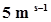to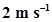. Calculate the work done by the force.

Ans. Work done by the force = change in kinetic energy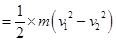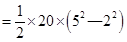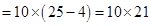= 210 J

###### 5. A mass of 10 kg is at a point A on a table. It is moved to a point B. If the line joining A and B is horizontal, what is the work done on the object by the gravitational force? Explain your answer.

Ans. Since work done on the object by gravitational force depends upon change in the vertical height of the object. Vertical height of the object is not changing as the joining A and B is horizontal at the same height hence the work done is zero.

NCERT Solutions for Class 9 Science Work and Energy part 2

###### 6. The potential energy of a freely falling object decreases progressively. Does this violate the law of conservation of energy? Why?

Ans. It doesn’t violate the law of conservation of energy because the potential energy of a freely falling object decreases progressively since it keeps changing into kinetic energy of the free falling object therefore the total energy to the object remains conserved.

###### 7. What are the various energy transformations that occur when you are riding a bicycle?

Ans. It is the transformation of our muscular energy into mechanical energy to ride the bicycle.

###### 8. Does the transfer of energy take place when you push a huge rock with all you might and fail to move it? Where is the energy you spend going?

Ans. While we push a huge rock with all our might(power) but fail to move it no energy transfer occurs as cellular energy simply wastes out in muscle contraction and relaxation even heat generation(sweating).

###### 9. A certain household has consumed 250 units of energy during a month. How much energy is this in joules?

Ans. Energy consumed by a certain household = 250 kWh

since 1 kWh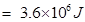therefore 250 kWh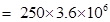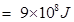NCERT Solutions for Class 9 Science Work and Energy part 2

###### 10. An object of mass 40 kg is raised to a height of 5 m above the ground. What is its potential energy? If the object is allowed to fall, find its kinetic energy when it is half way down.

Ans. Potential energy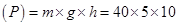= 2000 J

When the object is half way down the height of the object is = 2.5 m

initial velocity (u) = 0 (thrown from ground/rest)

since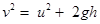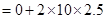= 50

Kinetic energy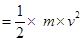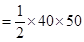= 1000 J

###### 11. What is the work done by the force of gravity on a satellite moving round the earth? Justify your answer.

Ans. The satellite is moving on a round path, displacement in the object is perpendicular to the direction of force.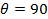0.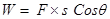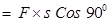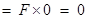Therefore, work done is zero.

NCERT Solutions for Class 9 Science Work and Energy part 2

Ans. No.

###### 13. A person holds a bundle of hay over his head for 30 minutes and gets tired. Has he done some work or not? Justify your answer.

Ans. When a person holds a bundle of hay over his head for 30 minutes and gets tired he applies force in upward direction and displacement of bundle of hay is in forward direction which is perpendicular to the direction of force applied therefore displacement is zero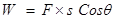No work done.

NCERT Solutions for Class 9 Science Work and Energy part 2

###### 14. An electric heater is rated 1500 W. How much energy does it use in 10 hours?

Ans. Electric heater’s power (p) = 1500 W = 1.5 kW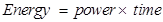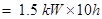= 15 kWh

###### 15. Illustrate the law of conservation of energy by discussing the energy changes which occur when we draw a pendulum bob to one side and allow it to oscillate. Why does the bob eventually come to rest? What happens to its energy eventually? Is it a violation of the law of conservation of energy?

Ans. The bob eventually comes to rest due to the frictional force offered by the air and the rigid support holding the thread.

It is not a violation of the law of conservation of energy since mechanical energy can get converted into another form of energy which cannot be utilised for useful work. This loss of energy is called dissipation of energy.

NCERT Solutions for Class 9 Science Work and Energy part 2

###### 16. An object of mass, m is moving with a constant velocity, v. How much work should be done on the object in order to bring the object to rest?

Ans. In order to bring the object to rest the work done must be equal to the kinetic energy of the moving object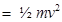###### 17. Calculate the work required to be done to stop a car of 1500 kg moving at a velocity of 60 km/h?

Ans. Initial velocity of the car (u) = 60 km/h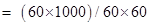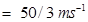Final velocity (v) = 0 (object has to be stopped)

Initial kinetic energy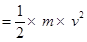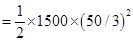= 208333.30 J

Final kinetic energy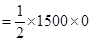= 0

Therefore, work done = change in kinetic energy = 208333.30 ― 0 = 208333.30 J

NCERT Solutions for Class 9 Science Work and Energy part 2

###### 18. In each of the following a force, F is acting on an object of mass, m. The direction of displacement is from west to east shown by the longer arrow. Observe the diagrams carefully and state whether the work done by the force is negative, positive or zero.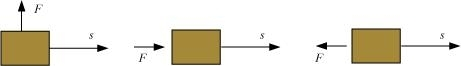Ans. (i) Since in this diagram displacement is perpendicular to the direction of force, so work done is zero.

(ii) Since in this diagram displacement is in the direction of force, so work done is positive.

(iii) Since in this diagram displacement is in the opposite direction of the force applied hence work done is negative.

###### 19. Soni says that the acceleration in an object could be zero even when several forces are acting on it. Do you agree with her? Why?

Ans. Yes we agree with her statement. Because when many balanced forces act on the object its displacement becomes zero.

###### 20. Find the energy in kW h consumed in 10 hours by four devices of power 500 W each.

Ans. Since Energy = power x time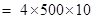= 20000 Wh = 20 kWh

NCERT Solutions for Class 9 Science Work and Energy part 2

###### 21. A freely falling object eventually stops on reaching the ground. What happens to its kinetic energy?

Ans. A free falling object eventually stops on reaching the ground since on striking the ground its kinetic energy is transmitted to the ground.

## NCERT Solutions for Class 9 Science

NCERT Solutions Class 9 Science PDF (Download) Free from myCBSEguide app and myCBSEguide website. Ncert solution class 9 Science includes text book solutions .NCERT Solutions for CBSE Class 9 Science have total 15 chapters. 9 Science NCERT Solutions in PDF for free Download on our website. Ncert Science class 9 solutions PDF and Science ncert class 9 PDF solutions with latest modifications and as per the latest CBSE syllabus are only available in myCBSEguide.

## CBSE app for Class 9

To download NCERT Solutions for class 9 Science, Computer Science, Home Science,Hindi ,English, Maths Social Science do check myCBSEguide app or website. myCBSEguide provides sample papers with solution, test papers for chapter-wise practice, NCERT solutions, NCERT Exemplar solutions, quick revision notes for ready reference, CBSE guess papers and CBSE important question papers. Sample Paper all are made available through the best app for CBSE students and myCBSEguide website.### Test Generator

Create question paper PDF and online tests with your own name & logo in minutes.### myCBSEguide

Question Bank, Mock Tests, Exam Papers, NCERT Solutions, Sample Papers, Notes